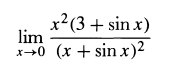# Limit of [x^2(3 + sinx)] / [(x + sinx)^2]

• PhizKid

## Homework Statement## The Attempt at a Solution

I expanded everything out and got [3x^2 + x^2*sin(x)] / [x^2 + 2sin(x) + sin^2(x)] and I tried splitting the problem but it didn't work because the denominator is always 0 when I try plugging in '0' for 'x.' I can't find any way to cancel out the denominator

You can't really 'cancel out' the denominator. You could try l'Hopital's rule or substituting a series expansion of sin(x). Or factoring x^2 out of the denominator and using a known limit like sin(x)/x.

Oops, sorry, I forgot to mention this is for an introductory Calc 1 course, so none of that l'Hopital stuff...

But what do you mean about substituting a series expansion?

Oops, sorry, I forgot to mention this is for an introductory Calc 1 course, so none of that l'Hopital stuff...

But what do you mean about substituting a series expansion?

I think my last suggestion about factoring x^2 out of the denominator is the best. I added that to my post in an edit. Try that.

So then I get:

[x^2(3 + sinx)] / [x^2(1 + 2sinx/x + sin^2x/x] and cancel out the x^2's:

(3 + sinx) / (1 + 2sinx/x + sin^2x/x)

Editl: Oh, I had made an algebra error. I got it now, thanks